#### Logical Operations for the Steane Code

Today, I want to discuss how to obtain a universal set of gates for the Steane code.

Previously, we discussed Gottesman's method for constructing the encoded $\bar{X}$ and $\bar{Z}$ logical operations for stabilizer codes.

import staccd = stac.CommonCodes.generate_code('[[7,1,3]]')cd.construct_logical_operators()print('encoded X =')stac.print_paulis(cd.logical_xs)print('encoded Z =')stac.print_paulis(cd.logical_zs)
encoded X =

$\displaystyle IIIIXXX$

encoded Z =

$\displaystyle ZZIIIIZ$

There are many other $7$-qubit Pauli operators that can act as $\bar{X}$ and $\bar{Z}$. A nicer choice is $\bar{X} = X_0X_1X_2X_3X_4X_5X_6,$ $\bar{Z} = Z_0Z_1Z_2Z_3Z_4Z_5Z_6.$

### Clifford gates

Recall that the Clifford group is generated from the operations $H, S, CX$. We will first show how to find the logical

The Hadamard gate is also transversal (no two qubit gate between the qubits of a single block). It is $\bar{H} = \otimes_{i=0}^6 H_i = H_0H_1H_2H_3H_4H_5H_6.$ Why do we know this works? We can check that $\bar{H}\bar{X}\bar{H}^\dagger = \bar{Z}$, and that $\bar{H}\bar{Z}\bar{H}^\dagger = \bar{X}$, which are the required relations that $\bar{H}$ must obey.

logicalH = stac.Circuit()for i in range(7):    logicalH.append('H', i)logicalH.draw(output='latex',scale=0.7)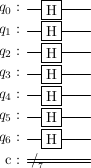#### $CX$ gate

The $CX$ gate is also transversal and actually just made of seven $CX$ gates. Let us have two logical qubits, and designate one as control and the other as target. Let the physical qubits of the control logical qubits be indexed by $(c,i)$ and those of the target logical qubit be indexed by $(t,i)$. Then $\bar{CX}_{c,t} = \otimes_{i=0}^6 CX_{(c,i),(t,i)}.$

To check that this works, we have to verify the conjgation relations that we had previously.

$G$ $CX_{01}GCX_{01}$
$X \otimes I$ $X \otimes X$
$I \otimes X$ $I \otimes X$
$Z \otimes I$ $Z \otimes I$
$I \otimes Z$ $Z \otimes Z$

Exercise: check that the $\bar{CX}$ satisfies these conjugation relations.

logicalCX = stac.Circuit()for i in range(7):    logicalCX.append('CX', i,7+i)logicalCX.draw(output='latex',scale=0.7)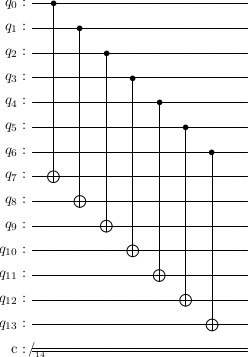#### Logical $S$ gate

The $S$ gate is the gate, $S = \begin{pmatrix}1 & 0 \\ 0 & \iu\end{pmatrix},$ but is often defined in a nicer fashion with an overall phase factor as $S = \begin{pmatrix}e^{-\iu\frac{\pi}{4}} & 0 \\ 0 & e^{\iu\frac{\pi}{4}}\end{pmatrix}.$

Exercise: Compute the conjugacy relations of $S$ i.e. $SGS^\dagger$ for $G=X,Y$.

Let $\bar{S} = \otimes_{i=0}^6 ZS.$

Exercise: Verify that the defined $\bar{S}$ satisfies the conjugacy relations that $S$ should have.

### The $T$ gate

The Clifford gate set does not allow us to do universal quantum computation, but the addition of the $T$ gate will. The $T$ gate is $S = \begin{pmatrix}1 & 0 \\ 0 & e^{i\frac{\pi}{4}}\end{pmatrix},$ or $S = \begin{pmatrix}e^{-\iu\frac{\pi}{8}} & 0 \\ 0 & e^{-\iu\frac{\pi}{8}}\end{pmatrix}.$

### Magic states

For the Steane code, it's not possible to create a transversal $T$ gate on an encoded qubit. The way to obtain the $T$ gate is via magic states. The relevant magic state in our case is $\ket{T} = T\ket{+} = \frac{\ket{0} + e^{i\frac{\pi}{4}}\ket{1}}{\sqrt{2}}.$ This state is used in the following circuit, where $\ket{\psi}=\alpha\ket{0} + \beta\ket{1}$ be some unknown state on which we want to apply the $T$ gate, i.e we want $T\ket{\psi} = \alpha\ket{0} + \beta e^{i\frac{\pi}{4}}\ket{1}$.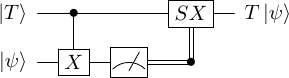Let's evaluate to make sure everything works. Starting with $\ket{T}\ket{\psi}$, if we apply the $CX$ gate from the first qubit to the second, we obtain, $CX_{01}\ket{T}\ket{\psi} = CX_{01}(\ket{0}+e^{i\frac{\pi}{4}}\ket{1})(\alpha\ket{0}+\beta\ket{1})/\sqrt{2},$ $= (\alpha\ket{00} + \beta\ket{01} + \alpha e^{i\frac{\pi}{4}}\ket{11} + \beta e^{i\frac{\pi}{4}}\ket{10})/\sqrt{2},$ $= \frac{1}{\sqrt{2}}(\alpha\ket{0} + e^{i\frac{\pi}{4}}\beta\ket{1})\ket{0} + \frac{1}{\sqrt{2}}(\beta\ket{0} + e^{i\frac{\pi}{4}}\alpha\ket{1})\ket{1}.$ Next, we measure the second qubit in the $Z$ basis. We either obtain $0$ and the first qubit is left in the state $\alpha\ket{0} + \beta e^{i\frac{\pi}{4}}\ket{1} = T\ket{\psi},$ or we obtain $1$ and the first qubit is left in the state $\beta\ket{0} + \alpha e^{i\frac{\pi}{4}}\ket{1}.$ In the first case, we have obtained the desired state. In the second case, if we just apply, $SX$ to the state, we obtain $T\ket{\psi}$.

In summary, if it is possible to create a $\ket{T}$ state, one can apply a $T$ gate to any state using just Clifford operations.

### Creating the $\ket{T}$ state

One can probabilistically create the $\ket{T}$ state. The circuit for it is given below, where the second control gate is a $CS$ gate.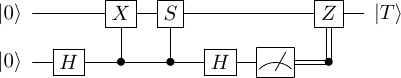Tstateprep = stac.Circuit()Tstateprep.append('H', 1)Tstateprep.append('CX', 1, 0)Tstateprep.append('cp(pi/2)', 1, 0)Tstateprep.append('H', 1)Tstateprep.append('M', 1)Tstateprep.draw()# an iteration that yielded 0 for the measurementTstateprep.simulate()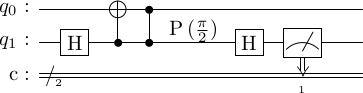basis  amplitude-------  -----------     00   0.707     10  0.707j
# an iteration that yielded 1 for the measurementTstateprep.simulate()
  basis  amplitude-------  -----------     01   0.707     11  -0.707j

Exercise: Evaluate this circuit by hand to show that if the measurement outcome is $0$, then the first qubit will be in the $\ket{T}$ state.

If instead the measurement outcome is $1$, then we apply the $Z$ operator to correct the state.